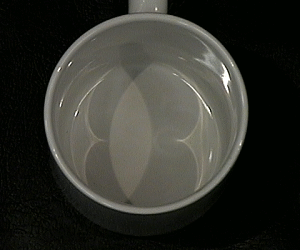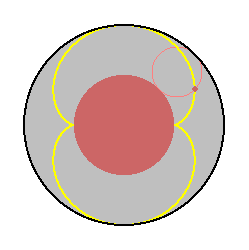# The Nephroid Lab

Welcome to The Nephroid Lab, an interactive laboratory in elementary computational algebraic geometry.

The immediate goal of this lab is to show that two curves defined by the pictures below are the same. Both are called the nephroid, because of their kidney shape.### Figure 1: An optical and mathematical nephroid curve.

The apparent curve in the first image is the pattern formed on the bottom of an empty flat-bottomed coffee mug when parallel light rays come in and bounce off the circular sides.

The similar looking curve in the second image is the path traced out by a point on the boundary of a small disk as the disk rolls along the boundary of a larger disk having twice the diameter.

In figuring out why the two nephroid curves are the same, we will encounter the following mathematical ideas:

• How to find a parametrization in terms of trigonometric functions for curves (like the nephroid) that may be generated by rolling one circle along the outside of another circle.
• How to convert trigonometric parametrizations into algebraic parametrizations.
• How to use elimination of variables to
• find implicit polynomial equations for parametrized curves
• compute profiles of projections of algebraic surfaces, profiles of surfaces, and envelopes of families of curves

## References and sources of inspiration

The following sources provided many of the ideas for this lab. One might also consult them for more precise formulations of the concepts it attempts to teach.
We also include here a link to some text describing the intended audience and usage for the lab.
Next: Parametrizing the Nephroid
The authors would like to thank Stuart Levy for helping to photograph the coffee cup nephroid on this title page.

Vic Reiner <reiner@math.umn.edu>
Frederick J. Wicklin <fjw@geom.umn.edu>